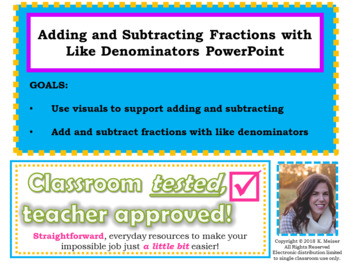# Adding and Subtracting Fractions with Like Denominators LessonSubject
Resource Type
File Type

Presentation (Powerpoint) File

(351 KB|9 pages)
Standards
• Product Description
• StandardsNEW
SHORT AND SWEET! This is a PowerPoint lesson to teach and show how to add and subtract fractions with like denominators. Best used with SMARTBoards (or equivalent) to allow students the opportunity to come to the board.

Students will...
*Visually see fraction bars used for addition and subtraction
*Use the algorithm to add and subtract fractions

Introduction to Fractions 2 Day Lesson
Equivalent Fractions- 2 Day Lesson
Compare and Order Fractions 3 Day Lesson
Mixed Numbers and Improper Fractions LessonMultiply Fractions by Whole Number LessonFractions of a Group-2 day PowerPoint LessonMixed Numbers on a Number Line
Multiplying Fractions on a Number LineFractional Line Plot Data
Understand addition and subtraction of fractions as joining and separating parts referring to the same whole.
Total Pages
9 pages
N/A
Teaching Duration
N/A
Report this Resource to TpT
Reported resources will be reviewed by our team. Report this resource to let us know if this resource violates TpT’s content guidelines.